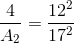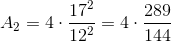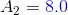## Example Questions

### Example Question #21 : Hexagons

Two hexagons are similar. If they have the ratio of 4:5, and the side of the first hexagon is 16, what is the side of the second hexagon?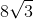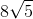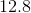Explanation:

This type of problem can be solved through using the formula: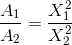If the ratio of the hexagons is 4:5, then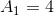and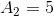.denotes area. The side length of the first hexagon is 16.

Substituting in the numbers, the formula looks like: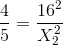This quickly begins a problem where the second area can be solved for by rearranging all the given information.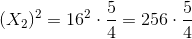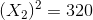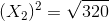The radical can then by simplified with or without a calculator.

Without a calculator, the 320 can be factored out and simplified: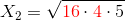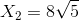### Example Question #22 : Hexagons

The ratio between two similar hexagons is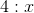. The side length of the first hexagon is 12 and the second is 17. What mustbe?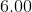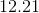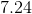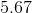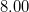Explanation:

This kind of problem can be solved for by using the formula:where thevalues are the similarity ratio and thevalues are the side lengths.

In this case, the problem provides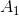, but not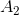.is denoted asin the question.

There are four variables in this formula, and three of them are provided in the problem. This means that we can solve for() by substituting in all known values and rearranging the formula so it's in terms of